2015年2月，Google在Nature上发表了一篇论文(见附件)：Human-level control through deep reinforcement learning。文章描述了如何让电脑自己学会打Atari 2600电子游戏。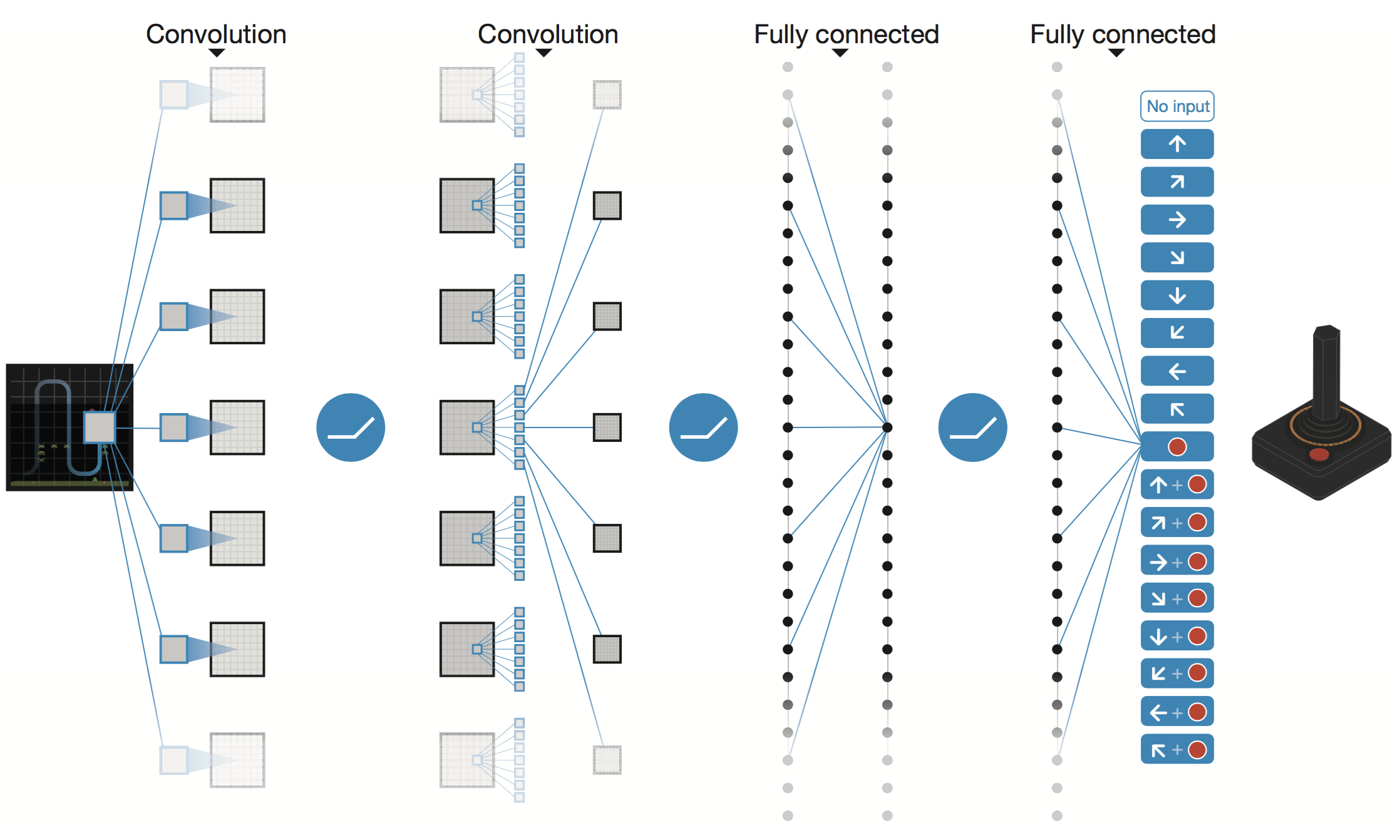2. 强化学习(Q-Learning)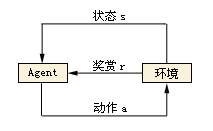2.1. 马尔可夫决策过程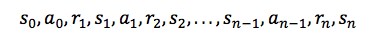2.2. 折扣未来奖励（Discounted Future Reward）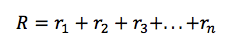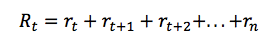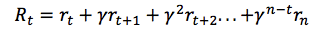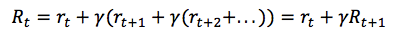2.3. Q-Learning算法描述：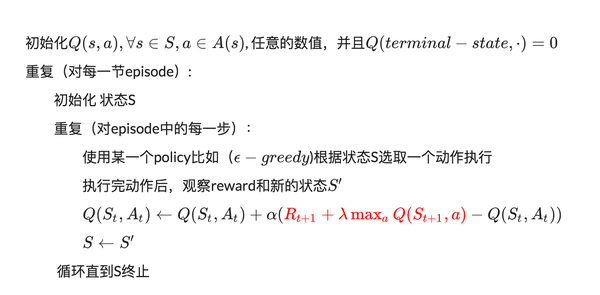3. 卷积神经网络(CNN)

3.1. 局部感知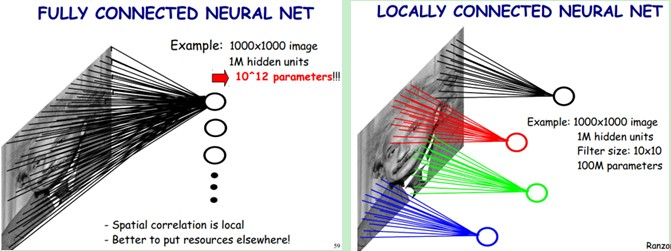3.2. 参数共享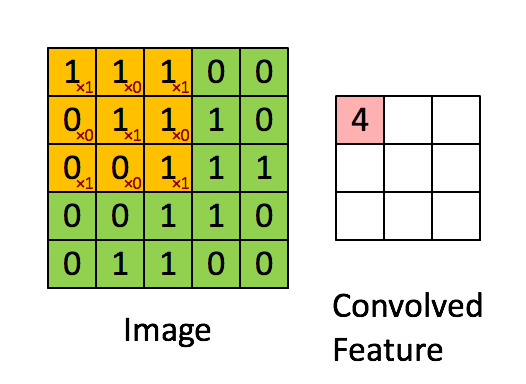3.3. 多卷积核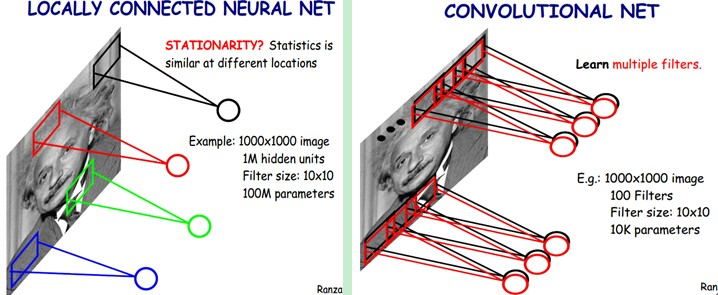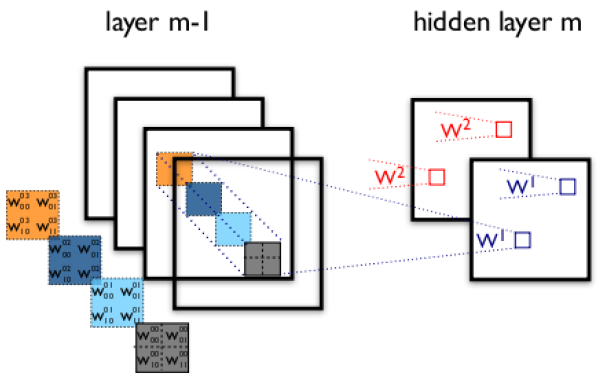3.4. Down-pooling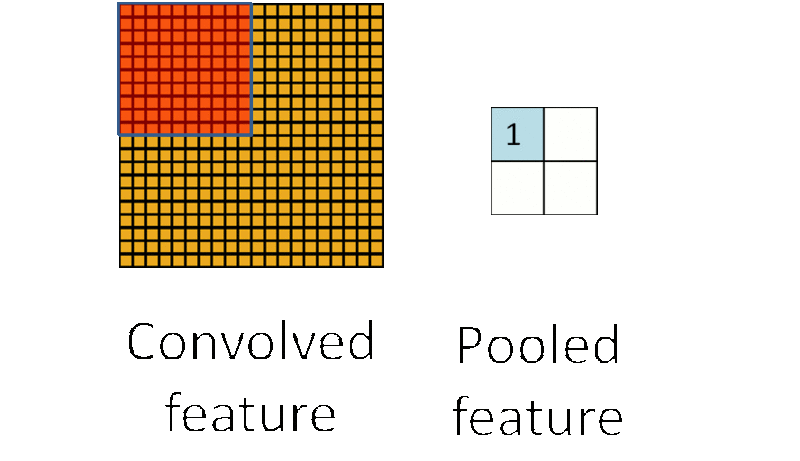4. DQN算法描述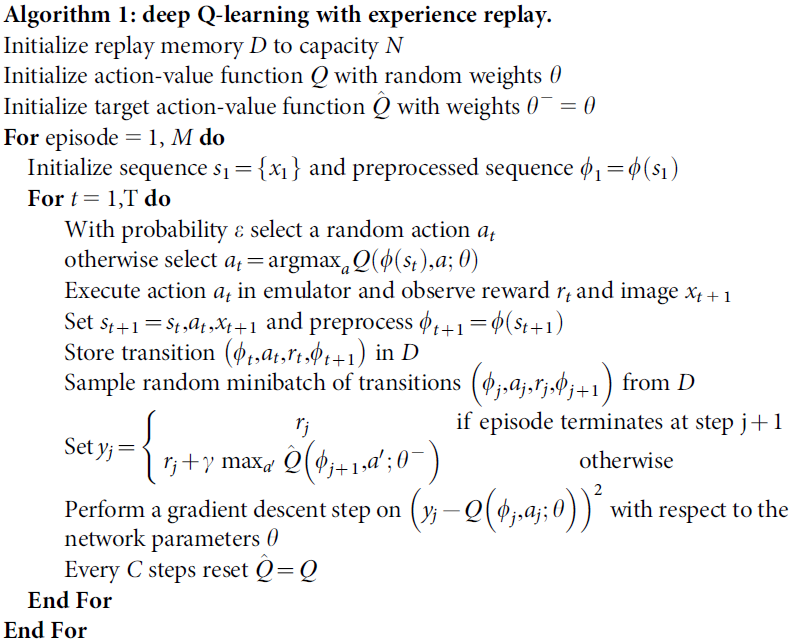5. 使用DQN训练“接砖块”游戏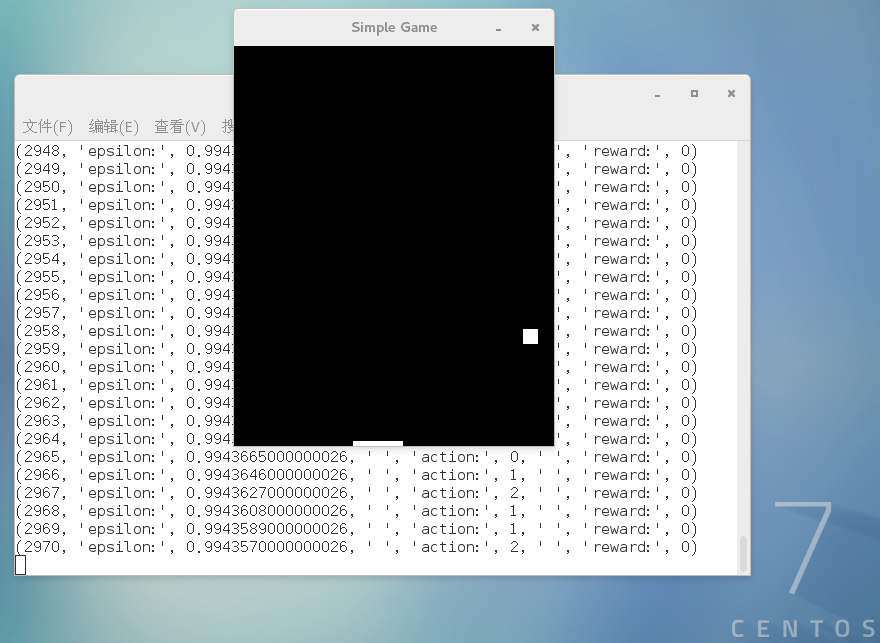#Game的定义类，此处Game是什么不重要，只要提供执行Action的方法，获取当前游戏区域像素的方法即可class Game(object):    def __init__(self):  #Game初始化
# action是MOVE_STAY、MOVE_LEFT、MOVE_RIGHT
# ai控制棒子左右移动；返回游戏界面像素数和对应的奖励。(像素->奖励->强化棒子往奖励高的方向移动)
def step(self, action):

# learning_rateLEARNING_RATE = 0.99# 跟新梯度
INITIAL_EPSILON = 1.0FINAL_EPSILON = 0.05# 测试观测次数
EXPLORE = 500000 OBSERVE = 500# 记忆经验大小
REPLAY_MEMORY = 500000# 每次训练取出的记录数
BATCH = 100# 输出层神经元数。代表3种操作-MOVE_STAY:[1, 0, 0]  MOVE_LEFT:[0, 1, 0]  MOVE_RIGHT:[0, 0, 1]
output = 3  # MOVE_STAY:[1, 0, 0]  MOVE_LEFT:[0, 1, 0]  MOVE_RIGHT:[0, 0, 1]
input_image = tf.placeholder("float", [None, 80, 100, 4])  # 游戏像素
action = tf.placeholder("float", [None, output])     # 操作
#定义CNN-卷积神经网络
def convolutional_neural_network(input_image):
weights = {'w_conv1':tf.Variable(tf.zeros([8, 8, 4, 32])),               'w_conv2':tf.Variable(tf.zeros([4, 4, 32, 64])),               'w_conv3':tf.Variable(tf.zeros([3, 3, 64, 64])),               'w_fc4':tf.Variable(tf.zeros([3456, 784])),               'w_out':tf.Variable(tf.zeros([784, output]))}

biases = {'b_conv1':tf.Variable(tf.zeros()),              'b_conv2':tf.Variable(tf.zeros()),              'b_conv3':tf.Variable(tf.zeros()),              'b_fc4':tf.Variable(tf.zeros()),              'b_out':tf.Variable(tf.zeros([output]))}

conv1 = tf.nn.relu(tf.nn.conv2d(input_image, weights['w_conv1'], strides = [1, 4, 4, 1], padding = "VALID") + biases['b_conv1'])
conv2 = tf.nn.relu(tf.nn.conv2d(conv1, weights['w_conv2'], strides = [1, 2, 2, 1], padding = "VALID") + biases['b_conv2'])
conv3 = tf.nn.relu(tf.nn.conv2d(conv2, weights['w_conv3'], strides = [1, 1, 1, 1], padding = "VALID") + biases['b_conv3'])
conv3_flat = tf.reshape(conv3, [-1, 3456])
fc4 = tf.nn.relu(tf.matmul(conv3_flat, weights['w_fc4']) + biases['b_fc4'])

output_layer = tf.matmul(fc4, weights['w_out']) + biases['b_out']    return output_layer

#训练神经网络
def train_neural_network(input_image):
predict_action = convolutional_neural_network(input_image)

argmax = tf.placeholder("float", [None, output])
gt = tf.placeholder("float", [None])

action = tf.reduce_sum(tf.mul(predict_action, argmax), reduction_indices = 1)
cost = tf.reduce_mean(tf.square(action - gt))

game = Game()
D = deque()

_, image = game.step(MOVE_STAY)
image = cv2.cvtColor(cv2.resize(image, (100, 80)), cv2.COLOR_BGR2GRAY)
ret, image = cv2.threshold(image, 1, 255, cv2.THRESH_BINARY)
input_image_data = np.stack((image, image, image, image), axis = 2)
#print ("IMG2:%s" %input_image_data)    with tf.Session() as sess:
sess.run(tf.initialize_all_variables())

saver = tf.train.Saver()

n = 0
epsilon = INITIAL_EPSILON        while True:
#print("InputImageData:", input_image_data)
action_t = predict_action.eval(feed_dict = {input_image : [input_image_data]})

argmax_t = np.zeros([output], dtype=np.int)            if(random.random() <= INITIAL_EPSILON):
maxIndex = random.randrange(output)            else:
maxIndex = np.argmax(action_t)
argmax_t[maxIndex] = 1
if epsilon > FINAL_EPSILON:
epsilon -= (INITIAL_EPSILON - FINAL_EPSILON) / EXPLORE

reward, image = game.step(list(argmax_t))

image = cv2.cvtColor(cv2.resize(image, (100, 80)), cv2.COLOR_BGR2GRAY)
ret, image = cv2.threshold(image, 1, 255, cv2.THRESH_BINARY)
image = np.reshape(image, (80, 100, 1))
input_image_data1 = np.append(image, input_image_data[:, :, 0:3], axis = 2)

D.append((input_image_data, argmax_t, reward, input_image_data1))            if len(D) > REPLAY_MEMORY:
D.popleft()            if n > OBSERVE:
minibatch = random.sample(D, BATCH)
input_image_data_batch = [d for d in minibatch]
argmax_batch = [d for d in minibatch]
reward_batch = [d for d in minibatch]
input_image_data1_batch = [d for d in minibatch]

gt_batch = []

out_batch = predict_action.eval(feed_dict = {input_image : input_image_data1_batch})                for i in range(0, len(minibatch)):
gt_batch.append(reward_batch[i] + LEARNING_RATE * np.max(out_batch[i]))

print("gt_batch:", gt_batch, "argmax:", argmax_batch)
optimizer.run(feed_dict = {gt : gt_batch, argmax : argmax_batch, input_image : input_image_data_batch})

input_image_data = input_image_data1
n = n+1
print(n, "epsilon:", epsilon, " " ,"action:", maxIndex, " " ,"reward:", reward)

train_neural_network(input_image)